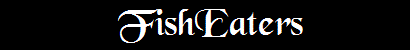``Where the Bishop is, there let the multitude of believers be; even as where Jesus is, there is the Catholic Church'' Ignatius of Antioch, 1st c. A.D

### Date of Easter

Easter will fall on the first Sunday after the first full Moon that falls on or after March 21 (not, as commonly belived, the "first Sunday after the first full Moon in Spring" where Spring is defined as the Season which begins with the astronomical vernal equinox).

The earliest possible date for Easter is March 22, and the latest possible date for it is April 25.

 2003 April 20 2004 April 11 2005 March 27 2006 April 16 2007 April 8 2008 March 23 2009 April 12 2010 April 4 2011 April 24 2012 April 8 2013 March 31 2014 April 20 2015 April 5 2016 March 27 2017 April 16 2018 April 1 2019 April 21 2020 April 12 2021 April 4 2022 April 17 2023 April 9 2024 March 31 2025 April 20

For the mathematically minded, there is an algorithm one can use to determine the date of Easter for any given year. In the following equations:

y=year of Easter
d=day of Easter
m=month of Easter

All variables are integers; drop all remainders from division operations:

c = y / 100

n = y - 19 * ( y / 19 )

k = ( c - 17 ) / 25

i = c - c / 4 - ( c - k ) / 3 + 19 * n + 15

i = i - 30 * ( i / 30 )

i = i - ( i / 28 ) * ( 1 - ( i / 28 ) * ( 29 / ( i + 1 ) ) * ( ( 21 - n ) / 11 ) )

j = y + y / 4 + i + 2 - c + c / 4

j = j - 7 * ( j / 7 )

l = i - j

m = 3 + ( l + 40 ) / 44

d = l + 28 - 31 * ( m / 4 )Back to The Liturgical Year I: IntroBack to Being CatholicIndex